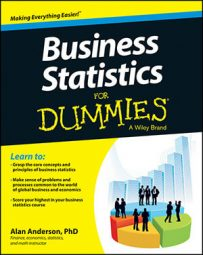When testing a hypothesis for a small sample where you have to find the appropriate critical right-tail value, this value depends on certain criteria. In addition to being positive, the value also depends on the sample size and whether or not the population standard deviation is known.

After you calculate a test statistic, you compare it to one or two critical values, depending on the alternative hypothesis, to determine whether you should reject the null hypothesis.

A critical value shows the number of standard deviations away from the mean of a distribution where a specified percentage of the distribution is above the critical value and the remainder of the distribution is below the critical value. A right-tailed test has one positive critical value.

A small sample is less than 30. When you use a small sample to test a hypothesis about the population mean, you take the resulting critical value from the Student's t-distribution. In a right-tailed test, the critical value is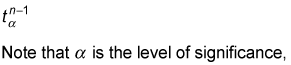and n represents the sample size.

You draw these critical values from the Student's t-distribution with n – 1 degrees of freedom (df).

The number of degrees of freedom used with the t-distribution depends on the particular application. For testing hypotheses about the population mean, the appropriate number of degrees of freedom is one less than the sample size (that is, n – 1).

The critical value or values are used to locate the area under the curve of a distribution that is too extreme to be consistent with the null hypothesis. For a right-tailed test, these are the large positive values, which are collectively known as the right tail of the distribution. The area in the tail equals the level of significance of the hypothesis test.

As an example of a right-tailed test, suppose the level of significance is 0.05 and the sample size is 10; then you get a single positive critical value: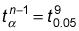Refer to the Student's t-distribution table to find the intersection of the row representing 9 degrees of freedom and the column headed t0.05.

The Student's t-distribution
Degrees of Freedom t0.10 t0.05 t0.025 t0.01 t0.005
6 1.440 1.943 2.447 3.143 3.707
7 1.415 1.895 2.365 2.998 3.499
8 1.397 1.860 2.306 2.896 3.355
9 1.383 1.833 2.262 2.821 3.250
10 1.372 1.812 2.228 2.764 3.169
11 1.363 1.796 2.201 2.718 3.106
12 1.356 1.782 2.179 2.681 3.055
13 1.350 1.771 2.160 2.650 3.012
14 1.345 1.761 2.145 2.624 2.977
15 1.341 1.753 2.131 2.602 2.947

The critical value is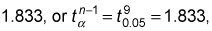as shown here.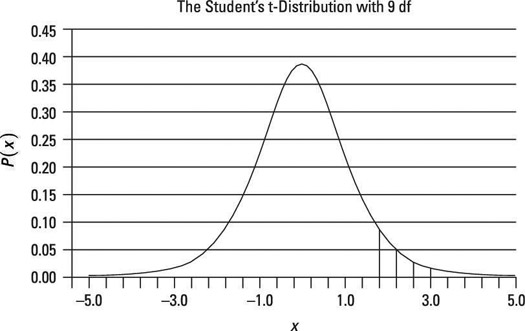Critical value taken from the t-distribution: right-tailed test.

The shaded region in the right tail represents the rejection region; if the test statistic falls in this area, the null hypothesis will be rejected.